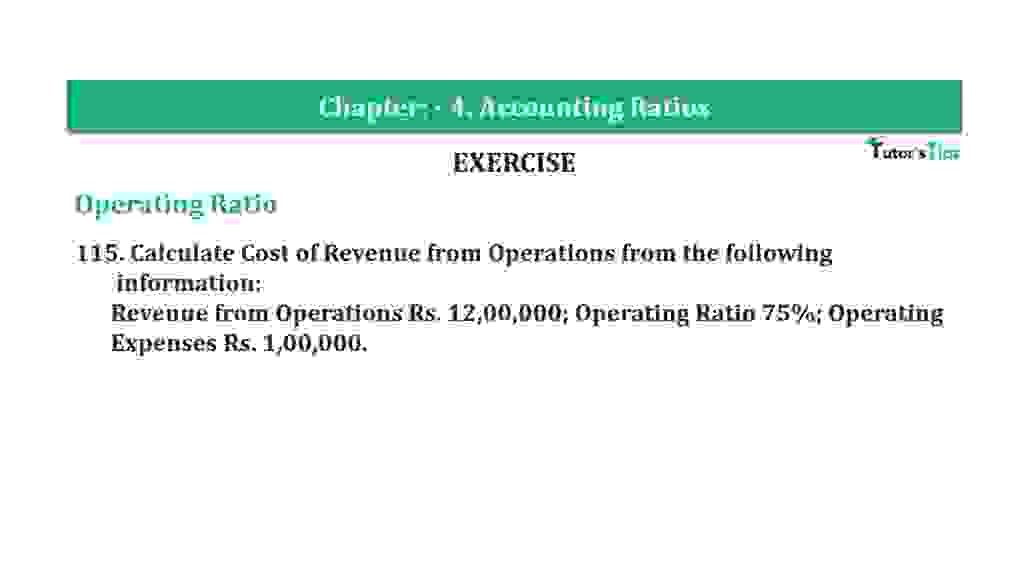# Question 115 Chapter 4 of +2-B – T.S. Grewal 12 ClassQuestion 115 Chapter 4 of +2-B

Operating Ratio

115. Calculate Cost of Revenue from Operations from the following
information: Revenue from Operations Rs. 12,00,000; Operating Ratio 75%; Operating Expenses Rs. 1,00,000.

### The solution of Question 115 Chapter 4 of +2-B: –

 Net Sales = Rs. 12,00,000 Operating Ratio = 75% Operating Expense = Rs. 1,00,000

 Operating Ratio = Operating Cost X 100 Net Sales
 75% = Operating Cost X 100 Rs. 12,00,000
 Operating Cost = Rs. 12,00,000 75% = Rs. 9,00,000

 Operating Cost = Cost of Revenue from Operations + Operating Expenses Rs. 9,00,000 = Cost of Revenue from Operations + Rs. 9,00,000 Cost of Revenue from Operations = Rs. 9,00,000 – Rs. 1,00,000 = Rs. 8,00,000

Balance Sheet: Meaning, Format & Examples

Thanks, Please Like and share with your friends

Comment if you have any question.

Also, Check out the solved question of previous Chapters: –

### T.S. Grewal’s Double Entry Book Keeping (Vol. II: Accounting for Companies)T.S. Grewal’s Analysis of Financial Statements